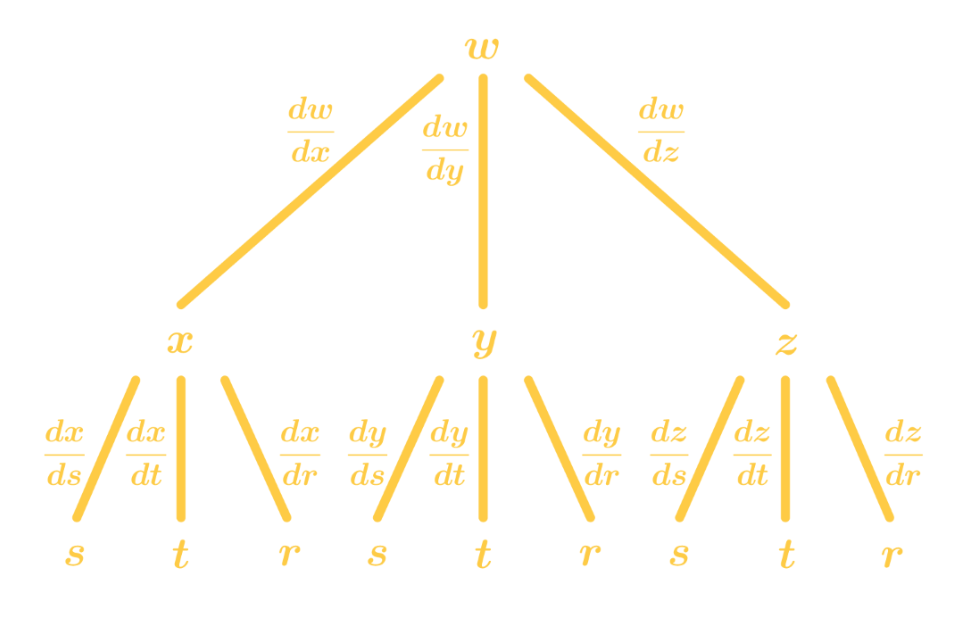# Chain rule for multivariable functions

### Chain rule for multivariable functions

#### Lessons

Notes:

Suppose we have a function $y=f(g(x))$. Then the chain rule is:

$y\ = f'(g(x))g'(x)$

We can rewrite this using an alternate notation:

$\frac{dy}{dx} = \frac{df}{dg} \frac{dg}{dx}$

Now if we were to change $g \to x$ and $x\to t$, then we have the chain rule to be:

$\frac{dy}{dt} = \frac{df}{dx} \frac{dx}{dt}$

Why do we want this alternate notation? Because it relates to the chain rule for 2 variable functions.

1st Case of Chain Rule for 2 Variable Functions
Suppose we have $z=f(x,y)$, $x=g(t)$, and $y=h(t)$, then the chain rule (derivative of $z$ in respect to $t$) is:

$\frac{dz}{dt} = \frac{df}{dx} \frac{dx}{dt} + \frac{df}{dy} \frac{dy}{dt}$

2nd Case of Chain Rule for 2 Variable Functions
Suppose we have $z=f(x,y)$,$x=g(s, t)$,$y=h(s, t)$, then there are 2 chain rules.
The derivative of $z$ in respect to $s$ is:

$\frac{dz}{dx} = \frac{df}{dx} \frac{dx}{dx} + \frac{df}{dy} \frac{dy}{ds}$

The derivative of $z$ in respect to $t$ is:

$\frac{dz}{dt} = \frac{df}{dx} \frac{dx}{dt} + \frac{df}{dy} \frac{dy}{dt}$

Using a Tree Diagram for Chain Rule
Tree diagrams are very useful when finding the chain rule for multivariable functions with more than 2 variables.
For example, suppose we have $w=f(x,y,z), x=g(s,t,r), y=h(s,t,r)$ and $z=i(s,t,r)$, and we want to find $\frac{dw}{dt}$.
We can write the tree diagram below like this:Then we will multiply all the connected derivatives, and sum them up to have:

$\frac{dw}{dt} = \frac{df}{dx} \frac{dx}{dt} + \frac{df}{dy} \frac{dy}{dt} + \frac{df}{dz} \frac{dz}{dt}$

• Introduction
Chain Rule for Multivariable Functions Overview:
a)
A Review of Chain Rule
• What is the chain rule?
• $h(x)=f(g(x))\to h' =f'(g(x))g'(x)$
• alternate notation: $\frac{dt}{dt} = \frac{df}{dx} \frac{dx}{dt}$

b)
1st Case of Chain Rule for 2 Variable Functions
• $z=f(x,y), x=g(t), y=h(t)$
• Then $\frac{dz}{dt} = \frac{df}{dx} \frac{dx}{dt} + \frac{df}{dy} \frac{dy}{dt}$
• An example

c)
2nd Case of Chain Rule for 2 Variable Functions
• $z=f(x,y), x=g(s, t), y=h(s, t)$
• $\frac{dz}{dx} = \frac{df}{dx} \frac{dx}{dx} + \frac{df}{dy} \frac{dy}{ds}$
• $\frac{dz}{dt} = \frac{df}{dx} \frac{dx}{dt} + \frac{df}{dy} \frac{dy}{dt}$
• An example

d)
Using a Tree Diagram for Chain Rule
• Easier to find the chain rule for multivariable functions
• What it looks like
• Couple Examples

• 1.
Finding the Chain Rule for 2 Variable Functions
Given that $z=\sin (xy), x= t^3, y=1-t$, find $\frac{dz}{dt}$.

• 2.
Given that $z= \ln (x^2+y^2), x=\sqrt{s^2+t^2}, y=2s+t$, find $\frac{dz}{ds}$.

• 3.
Finding the Chain Rule for Implicit Functions
Given that $xy^2+ \cos (x^2 y)=3$, find $\frac{dy}{dx}$.

• 4.
Given that $e^{xz}+ \ln (xy)=x^2y^3$, find $\frac{dz}{dx}$.

• 5.
Using the Tree Diagram to get the Chain Rule
Suppose $w=f(x, y, z), x=g(s, t), y=h(s, t)$, $z=i(s, t)$. Use the tree diagram and use the chain rule to find $\frac{dw}{dt}$.

• 6.
Suppose $w=f(x, y, z), x=g(r, s, t), y=h(r, s, t), z=h(r, s, t), r=a(q).$ Use the tree diagram and use the chain rule to find $\frac{dw}{dq}$.